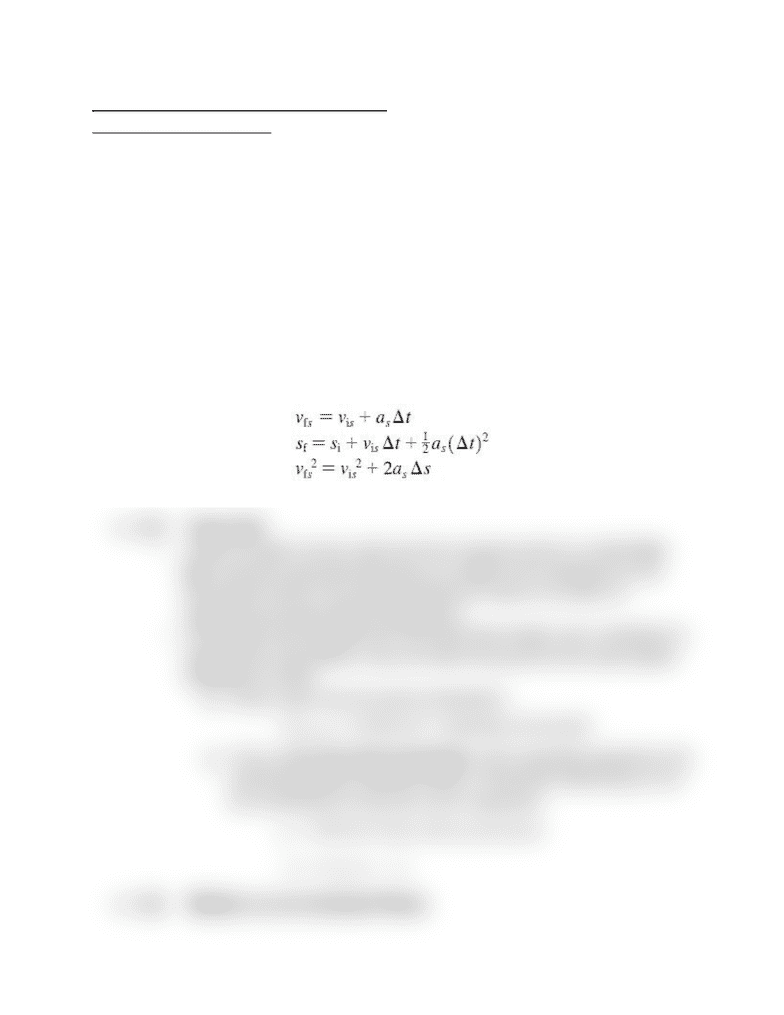Class Notes (1,100,000)
CA (650,000)
UTSC (30,000)
Lecture 5

# PHYA11H3 Lecture Notes - Lecture 5: Free Fall, Kinematics, One DirectionPremium

Department
Physics and Astrophysics
Course Code
PHYA11H3
Professor
Gyula Lorincz
Lecture
5

This preview shows half of the first page. to view the full 2 pages of the document.Introduction to Physics: Lecture 5
Physics I for Life Sciences
Chapter 2: Kinematics in One Dimension
Whats is Kinematics?
2.2. Finding Position from Velocity
Thus far, the three kinematics equations that describe an objects motion
with constant acceleration are as follows. Remember each equation can
be derived from the second equation (which we dont need to know how to
be able to do for this course) :
2.5. Free Fall
When an object moves under the force of gravity alone it is called free
fall. Free fall is assumed that there is no effect of air resistance on the
downwards motion of an object( because its value is so small, its
negligible and doesnt affect calculations)
If two object sare dropped from the exact same height, and ir resistance is
negated from calculations, they will reach the ground at the same speed,
regardless of mass.
Hence, they have the same acceleration:
This is called free fall acceleration, and is specially denoted by the
symbol g

(always a positive value). It should be noted that g is not
the acceleration by free fall, but its magnitude
2.6. Motion on an Inclined Plane